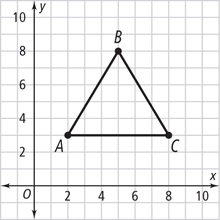# The perimeter 12

The perimeter of an equilateral triangle is 41.46 inches.

If the length of each side is (q - 5.7), what is the value of q?

q =  19.52 in

### Step-by-step explanation:Did you find an error or inaccuracy? Feel free to write us. Thank you!

Tips for related online calculators
Do you have a linear equation or system of equations and looking for its solution? Or do you have a quadratic equation?
Do you want to convert length units?
Calculation of an equilateral triangle.